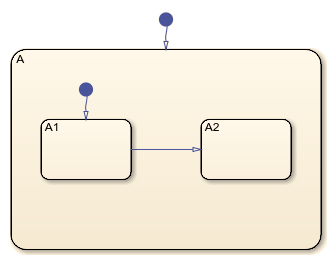# Create Charts by Using a MATLAB Script

This example shows how to include Stateflow® API commands in a MATLAB® function or script. Creating a script of API commands allows you to avoid repetitive chart creation steps and recreate the same model with a single command. For more information, see Overview of the Stateflow API.

### Run the MATLAB Function

The function `makeMyModel`, which is defined at the bottom of this page, produces a "base" Stateflow chart that you can reuse as a template for your applications.

```ch = makeMyModel; view(ch)```### Create Base Chart Function

This function creates a Stateflow chart and returns the corresponding `Stateflow.Chart` object.

`function ch = makeMyModel`

Create model and access new `Stateflow.Chart` object.

``` rt = sfroot; prev_machines = find(rt,"-isa","Stateflow.Machine"); sfnew; curr_machines = find(rt,"-isa","Stateflow.Machine"); m = setdiff(curr_machines,prev_machines); ch = find(m,"-isa","Stateflow.Chart");```

Create state `A` in chart.

``` sA = Stateflow.State(ch); sA.Name = "A"; sA.Position = [50 50 310 200];```

Create state `A1` inside of state `A`.

``` sA1 = Stateflow.State(ch); sA1.Name = "A1"; sA1.Position = [80 120 90 60];```

Create state `A2` inside of state `A`.

``` sA2 = Stateflow.State(ch); sA2.Name = "A2"; sA2.Position = [240 120 90 60];```

Create transition from `A1` to `A2`.

``` tA1A2 = Stateflow.Transition(ch); tA1A2.Source = sA1; tA1A2.Destination = sA2; tA1A2.SourceOClock = 3; tA1A2.DestinationOClock = 9;```

Add default transition to state `A`.

``` dtA = Stateflow.Transition(ch); dtA.Destination = sA; dtA.DestinationOClock = 0; dtA.SourceEndPoint = dtA.DestinationEndpoint-[0 30]; dtA.MidPoint = dtA.DestinationEndpoint-[0 15];```

Add default transition to state `A1`.

``` dtA1 = Stateflow.Transition(ch); dtA1.Destination = sA1; dtA1.DestinationOClock = 0; dtA1.SourceEndPoint = dtA1.DestinationEndpoint-[0 30]; dtA1.MidPoint = dtA1.DestinationEndpoint-[0 15]; end```